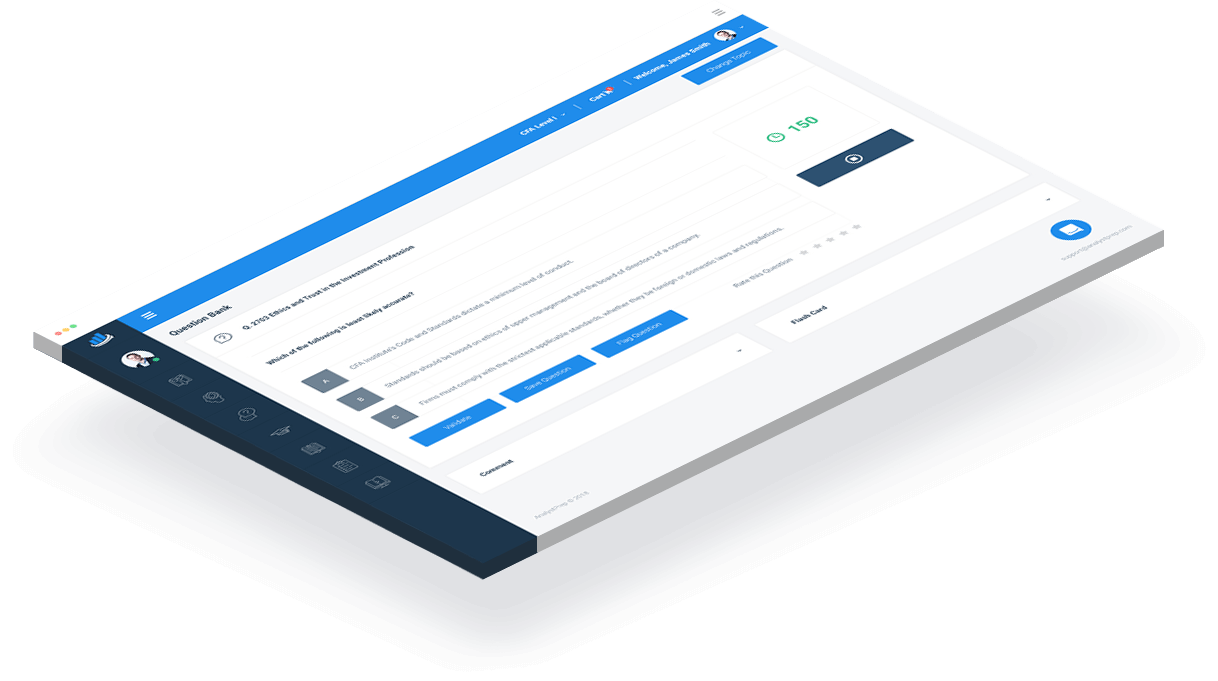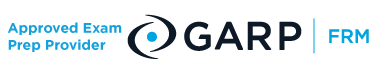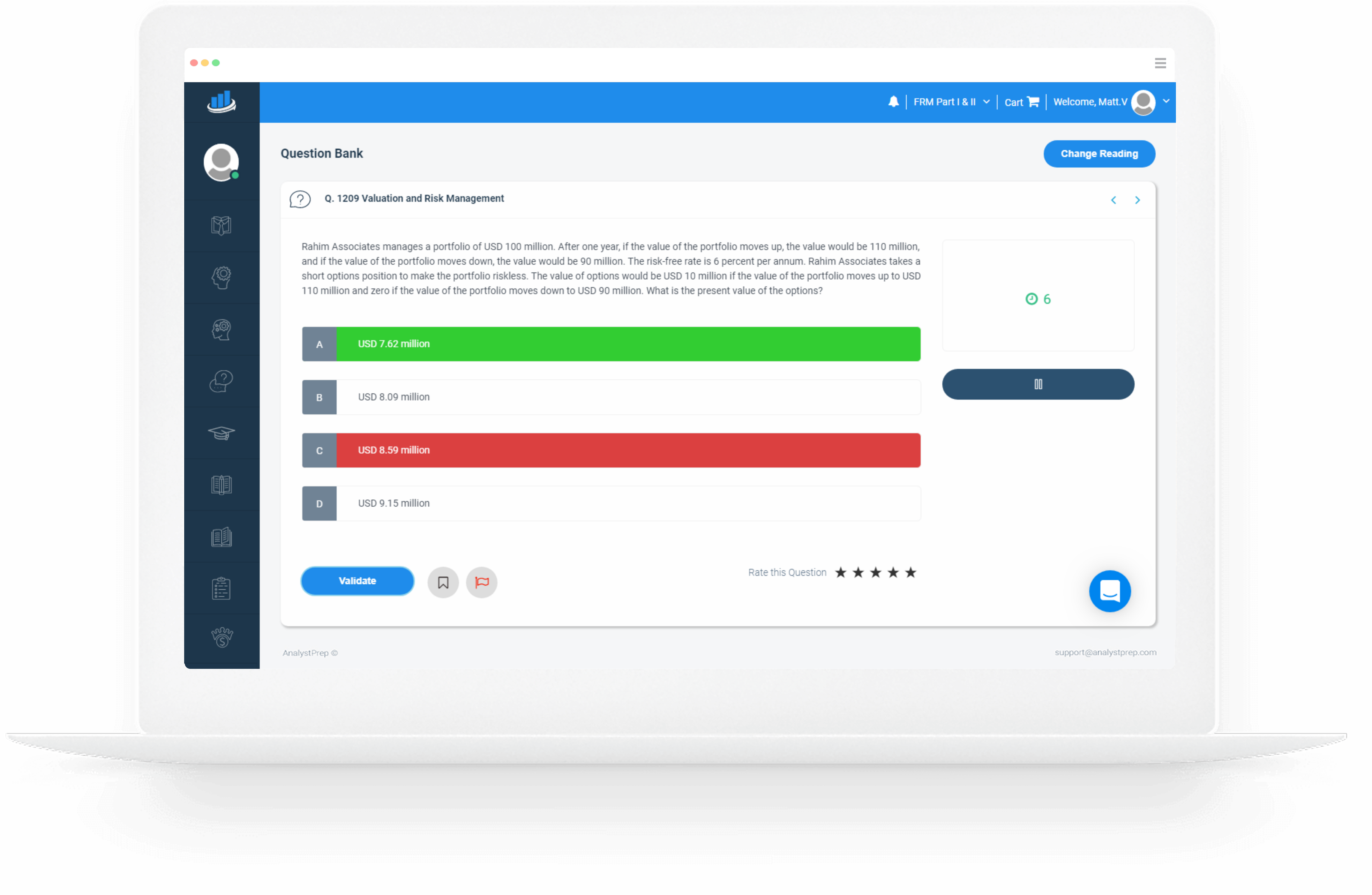Study Materials for 2023 CFA®, FRM®, Actuarial, GMAT® and EA® Exams

### FRM Part II Practice Questions

#### Only Hard, High-Quality Questions# There are no Shortcuts – Practice is Key

Our FRM Part II practice questions are designed to strengthen your understanding of key concepts and gauge your preparation with respect to your peers around the world.

Each question in the FRM Part II QBank has been developed by certified FRMs to ensure the highest level of quality and relevance. As a sign of trust, you get exclusive access to hundreds of FRM Part II practice questions as soon as you sign up. Thousands of candidates have used the question bank, with over 1 million questions answered.

Our practice questions are updated every year to ensure consistency with the most recent curriculum outlined by the GARP.

AnalystPrep’s game-changing algorithms enable you to compare your scores with those of other candidates around the globe.### Why AnalystPrep?

Our FRM part II practice questions are known not just for their level of detail but also for their high level of complexity. Preparing using too easy questions is a sure way to get frustrated on the big day, and candidates on feedback forums share as much. At AnalystPrep, we pride ourselves on thorough, continuously challenging questions that only make you a better-prepared candidate.

Our practice questions are subdivided according to readings to help you focus on a particular area at a time. If you need to gauge your knowledge on multiple readings, you can easily create a customized quiz with any number of questions.

We also include a comments section in each question through which you can link up with our experienced instructor for extra tips and tricks.

# Free FRM Part 2 Practice Questions

## Market Risk Measurement and Management

Jason Tyler has invested $100,000 in the shares of Kraken Corp. To calculate the market risk of his portfolio, Tyler gathers the monthly returns for the security over the last 500 months. The ten worst returns during this period were as follows: -19%, -24%, -27%, -23%, -20%, -16%, -21%, -22%, -18%, -30% What is the monthly VaR for Tyler’s investment using a confidence level of 99%? A)$16,000

B) $22,000 C)$21,000

D) $20,000 The correct answer is: C). In general, if there are n ordered observations, and a confidence level cl%, the cl% VaR is given by the $$[(1 – cl \%) n + 1]th$$ highest observation. This is the observation that separates the tail from the body of the distribution. For instance, if we have 1,000 observations and a confidence level of 95%, the 95% VaR is given by the (1 – 0.95)1,000 + 1 = 51st observation. There are 50 observations in the tail. In this case, the order (from highest to the lowest) are as follows: -30%, -27%, -24%, -23%, -22%, -21%, -20%, -19%, -18%, -16% The look-back window has 500 observations, so the 99% VaR is given by the (1 – 0.99)500 + 1 = 6th worst observation. Thus, monthly VaR = 21% *$100,000 = 21000 A is incorrect. The result is obtained by taking the sixth unordered observation as given in the question, i.e., -20% B is incorrect. The result is obtained by calculating the 99% VaR as the 5th worst observation, i.e., (1 – 0.99)500 D is incorrect. The result is obtained by taking the 5th unordered observation as given in the question, i.e., -20% ## Question 2 ## Credit Risk Measurement and Management On the table below are bid-ask quotes by ABC for CDS spreads for companies X, Y, and Z. CFC has excessive credit exposure to company Z. Analysts at CFC are looking to reduce this exposure by leveraging the CDS market. $$\begin{array}{c|c|c|c} & \textbf { 1 year } & \textbf { 3 years } & \textbf { 5 years } \\ \hline \mathrm{X} & \frac{10}{15} & \frac{22}{33} & \frac{28}{34} \\ \hline \mathrm{Y} & \frac{42}{55} & \frac{70}{99} & \frac{110}{133} \\ \hline \mathrm{Z} & \frac{68}{85} & \frac{92}{112} & \frac{138}{165} \end{array}$$ Because the farthest maturity of its exposure to Z is three years, CFC decides to buy a CAD 400 million three-year protection on Z from ABC. In a bid to make the purchase of this protection cheaper, based on professional judgment on X and Y, CFC decides to sell CAD 600 million five-year protection on X and to sell CAD 200 million one-year protection on Y to ABC. Determine the net annual premium payment made by CFC to ABC in the first year. A) CAD 0.54 million B) CAD 1.96 million C) CAD 1.5 million D) CAD 0.5 million The correct answer is: B). ABC is the market maker in these transactions. The table gives bid-ask quotes in basis points. The bid price is the amount that the market maker is prepared to spend to purchase CDS protection and the ask (offer) price is the amount the market maker is prepared to accept to sell such protection. In the first transaction, ABC is willing to sell protection to CFC with respect to Z at 112 basis points per year. Thus, the annual premium payable by CFC is CAD 4,480,000 ($$= \frac{112}{10,000}\times\ \text{CAD}\ 400,000,000$$). Recall that 1 basis point = 0.01%. In the second transaction, ABC will buy the protection offered by CFC at 28 basis points per year. Thus, the annual premium receivable by CFC is CAD 1,680,000 ($$= \frac{28}{10,000}\times \ \text{CAD}\ 600,000,000$$). In the final transaction, ABC will buy the protection offered by CFC at 42 basis points per year. Thus, the annual premium receivable by CFC is CAD 840,000 ($$= \frac{42}{10,000}\times \ \text{CAD}\ 200,000,000$$). Thus, $$\text{Net Annual Premium} = 4,480,000 – 1,680,000 – 840,000 =\text{CAD}\ 1,960,000\ \text{or CAD}\ 1.96\ \text{million}$$ ## Question 3 ## Credit Risk Measurement and Management Due to punitive international sanctions against country X over the use of banned weaponry, a company based in country X is facing the risk of an imminent rating downgrade. The following table gives the updated default probabilities for an A-rated issue by the company: $$\begin{array}{ll} \textbf{ Year } & \textbf { Default probability } \\ 1 & 0.400 \% \\ 2 & 0.500 \% \\ 3 & 0.600 \% \end{array}$$ Assuming that defaults can only occur at the end of the year, calculate the cumulative default rate at the end of each of the next three years. A) 4%, 0.750%, 1.235% B) 4%, 0.898%, 1.000% C) 4%, 0.750%, 0.980% D) 4%,0.898%,1.493% The correct answer is: D). Let: • $$d_t$$ denote the probability of default by the end of year $$t$$. • $$s_t$$ denote the probability of survival to the end of year $$t$$ • $$C_t$$ denote the cumulative default rate to the end of year $$t$$ Therefore, \begin{align} d_1 &= 0.400\%\\ d_2 &= 0.500\%\\ d_3 &= 0.600\%\end{align} The probability of survival to the end of year 1, $$S_1 = 1 – d_1 = 1 – 0.004 = 0.996$$ The cumulative default rate at the end of year 1, $$C_1 = 1 – S_1 = 1 – 0.996 = 0.004$$ The probability of survival to the end of year 2, $$S_2 = S_1 \times (1 – d_2) = 0.996(1 – 0.005) = 0.99102$$ The cumulative default rate to the end of year 2, $$C_2 = 1 – S_2 = 1 – 0.99102 = 0.00898$$ The probability of survival to the end of year 3, $$S_3 = S_2 \times (1 – d_3) = 0.99102 \times (1 – 0.006) = 0.98507$$ The cumulative default rate to the end of year 3, $$C_3 = 1 – S_3 = 1 – 0.98507 = 0.014926$$ ## Question 4 ## Liquidity and Treasury Risk Measurement and Management Samar Sarkar Brokerage specializes in providing margin loans to U.S. hedge funds that intend to buy securities on margin. One of the brokerage’s clients, Xenon Hedge Fund, wants to take a &dollar;3,000,000 equity position while putting up the minimum equity amount required by the Federal Reserve. Determine the margin loan amount and the leverage ratio of this position. A) Margin Loan3,000,000; Leverage Ratio 1.0

B) Margin Loan $1,500,000; Leverage Ratio 1.0 C) Margin Loan$1,500,000; Leverage Ratio 2.0

D) Margin Loan $3,000,000; Leverage Ratio 0.0 The correct answer is: C). According to Regulation T of the Federal Reserve Board, one may borrow up to 50 percent of the purchase price of securities that can be purchased on margin. Therefore, the margin loan will be$1,500,000 ($$= \3,000,000 \times 50\%$$). The remaining $1,500,000 has to be financed by equity. The leverage ratio is calculated as total assets divided by equity. Therefore, the hedge fund’s leverage ratio is 2.0 ($$= \frac{\3,000,000}{\1,500,000}$$). Note: By buying on margin, you borrow money from a broker. Margin accounts increase an investor’s purchasing power and allow them to use other people’s money to increase financial leverage. ## Question 5 ## Credit Risk Measurement and Management An investor has invested$10 million in a bond issued by Groningen Bank, based in Dortmund, Germany. He is acutely aware of the possibility of the bank defaulting and thus purchases a credit default swap from Bundesliga Bank, based in Munich, Germany. If the default probability of TVR and the default correlation between Groningen Bank and Bundesliga Bank increased, which of the following scenarios would play out?

A) The value of the CDS will increase, and the investor has wrong-way risk with Bundesliga Bank

B) The value of the CDS will decrease, and the investor has wrong-way risk with Bundesliga Bank

C) The value of the CDS will increase, and the investor has right-way risk with Bundesliga Bank

D) The value of the CDS will decrease, and the investor has right-way risk with Bundesliga Bank

The value of the CDS is mainly determined by the default probability of the reference entity, in this case, Groningen Bank. However, it’s also determined by the joint default correlation of Groningen Bank and Bundesliga Bank. Since the default probability of the reference entity has increased, the CDS spread widens. With the increase in correlation between Groningen and Bundesliga increasing, the present value of the CDS for the investor would fall. The investor also has a wrong-way correlation risk. The exposure to Groningen increases, and it’s more unlikely that Bundesliga Bank will pay the default insurance.

## Operational Risk and Resiliency

I. The customer appears unconcerned about the fees.

II. The address given by the customer and the branch where he came to open the account are not close to each other.

IV. The customer wants to open both a commercial account and a stock trading account.

A) I, II, and IV

B) I, II, and III

C) II, III, and IV

D) I,II,III and IV

It is apparent the customer is most likely not interested in trading stocks because he has very few questions about investing. What’s more, he doesn’t seem interested in finding out what the charges are, a typical question one would expect from a typical trader intent on keeping a large share of their profits. That he has traveled such a large distance to open an account at a faraway branch also raises questions because there likely could have been other banks closer to the address given, offering similar services.

However, there would be nothing wrong with opening multiple accounts – as long as the client’s identification documents are authentic and the purpose for each account is apparent.

## Credit Risk Measurement and Management

James works in a large investment. Recently, a new fund manager joined the team. Through a friend, who also happens to be a former colleague of the newly hired manager, James finds out that the new fund manager was adversely mentioned in a case involving accounting fraud in his previous firm, but details of the incident were not available in the public domain. James’s firm has a robust recruitment process, and part of its policy entails detailed background checks on all prospective employees. James assumes that the firm’s recruitment team must have looked into the manager’s records, and thus he does not feel compelled to disclose this information to the head of recruitment. He is also wary of damaging his relationship with the new fund manager. Select the most appropriate statement:

A) James must not disclose this information as it is not publicly available.

B)  James must disclose this information only if the new fund manager tries to commit fraud

C) James must not disclose this information as the fraud was committed at another firm

D) James must disclose this information to his team headOur FRM® study packages start as low as $249 with a one exam Practice package. Our Learn + Practice packages include study notes and video lessons for$399.